## TL;DR and Preamble

TL;DR: It is not always necessary to trade bias for variance when increasing model complexity.

Preamble: the charge of the electron, Millikan’s experiment, and anchoring
Richard Feynman made an insightful observation about the history of measurements of the charge of the electron: Millikan’s first measurement was noticeably lower than the (now known) true value. Over the next few decades, other groups produced estimates of the charge of the electron. Interestingly, almost all of them underestimated the true value (see Stack Exchange thread for more info). According to Feynman’s account, the subsequent measurements were biased by Millikan’s original measurement. This is a specific example of the cognitive bias known as anchoring.

Anchoring causes us to use an initial piece of information as an "anchor" that draws our interpretation of later information toward the anchor. This human tendency is not necessarily bad. It makes perfect sense from a Bayesian perspective where one tries to make the best use of finite resources (Lieder et al., 2012), but it can have negative consequences. The intuitive concept of the bias-variance tradeoff that is commonly taught has acted as a strong anchor in the machine learning community. We can see it incorrectly biasing the interpretation of experiments in Geman et al. (1992)'s landmark bias-variance tradeoff paper. It's time to cut this anchor loose.

Note: Because this is a blog post, it will be light on details. For many more details, please see the featured papers: On the Bias-Variance Tradeoff: Textbooks Need an Update (MSc Thesis) (Neal, 2019) and A Modern Take on the Bias-Variance Tradeoff in Neural Networks (Neal et al., 2018).

Stuart Geman, the man who influenced Steve Jobs’ uniform clothing style (according to a shaky Wikipedia contribution), gave us a concise statement of the bias-variance tradeoff:

The price to pay for achieving low bias is high variance (Geman et al., 1992).

In other words, when our model is too simple, it will have high bias and low variance; in order to achieve low bias, we can increase the complexity of the model, but this will inevitably result in high variance. This concept known as the bias-variance tradeoff is commonly illustrated with this graph (Fortmann-Roe, 2012):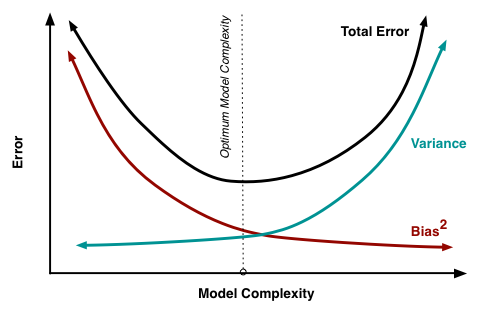The U-shaped test error curve is a key consequence of the bias-variance tradeoff, as it means that there is an optimal model complexity that perfectly balances bias and variance.

There is a fair amount of evidence for the bias-variance tradeoff in nonparametric models. For example, there is evidence of a bias-variance tradeoff in k-nearest neighbors (when varying k) and in kernel regression (when varying kernel width $\sigma$) (Geman et al., 1992):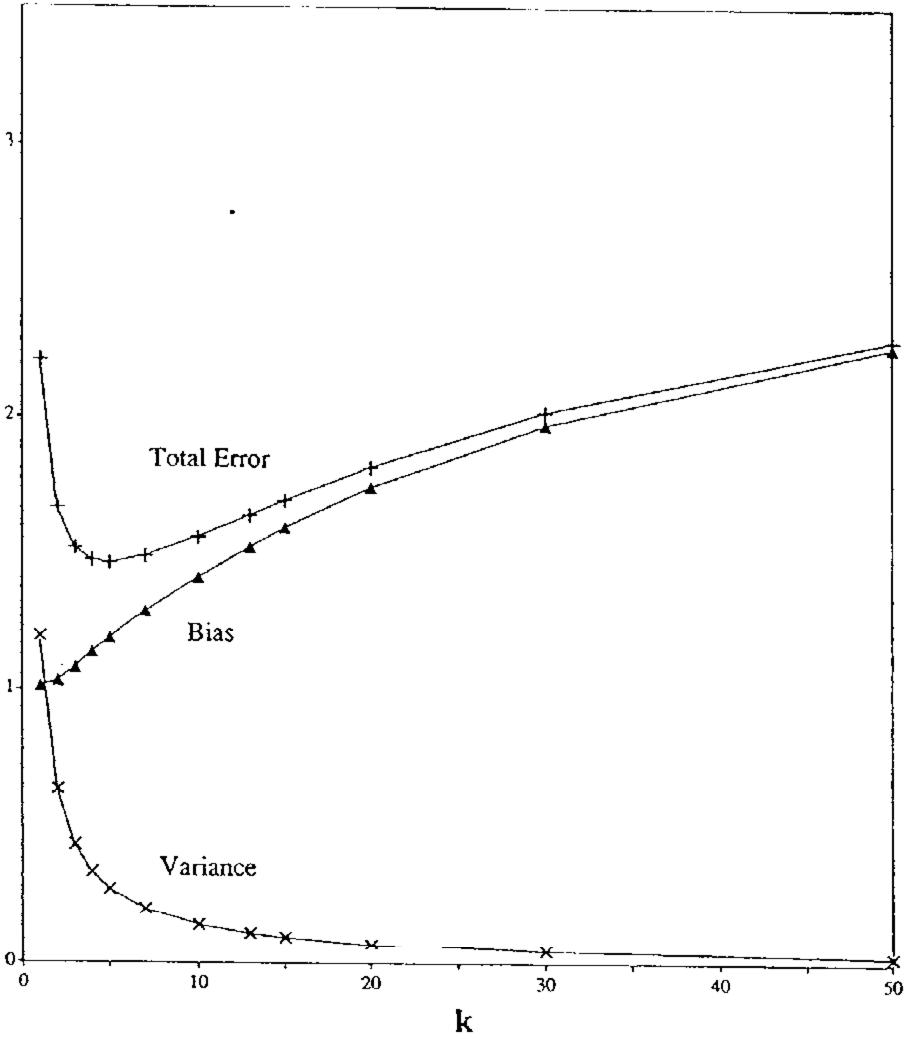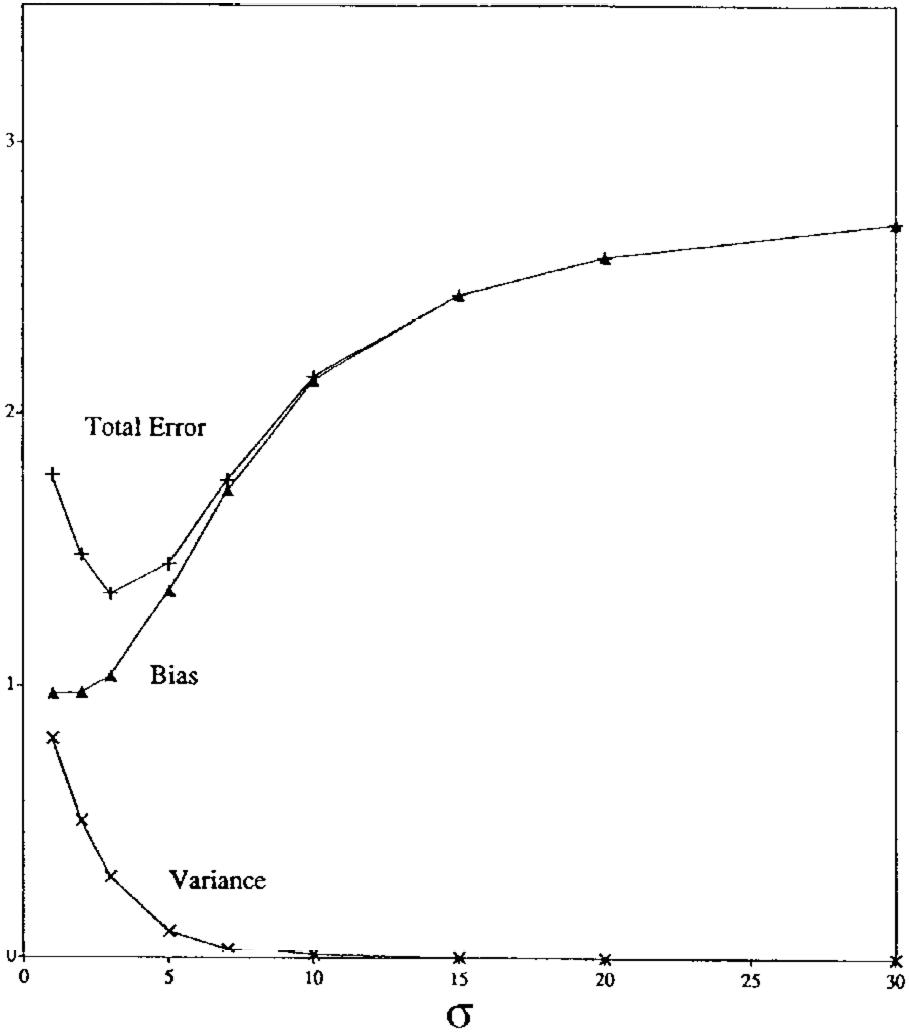Note that model complexity decreases with increasing k and $\sigma$, so the x-axis is reversed in these two graphs compared to the usual bias-variance tradeoff graph above.

For more examples of where we see the bias-variance tradeoff and more background on why we believe the bias-variance tradeoff, see Section 3.4 “Why do we believe the Bias-Variance Tradeoff?” of my MSc thesis.

## Motivation for Revisiting this Tradeoff

### Test Error Decreasing with Network Width

Despite the U-shape test error curve that the bias-variance tradeoff implies, Neyshabur et al. (2015) actually found that test error simply decreases with network width: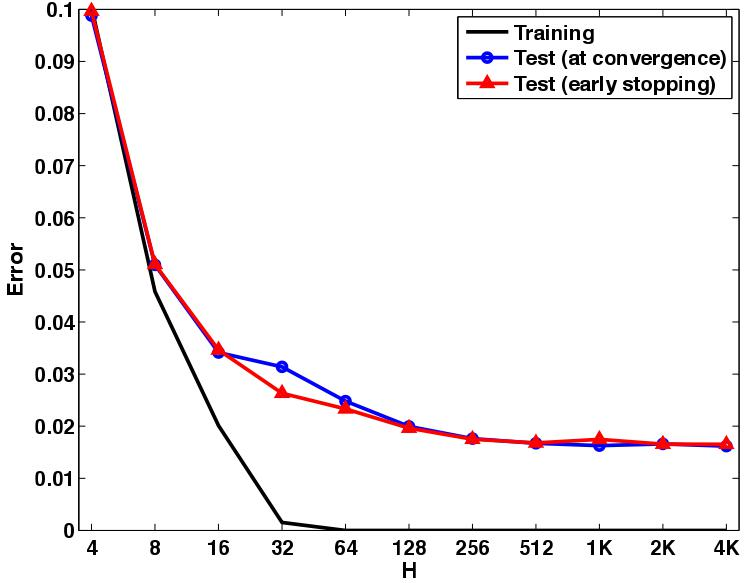There has been a large amount of supporting evidence for this finding since 2015, with some extra detail such as the “double descent” curve, which we briefly cover further below.

### Red Flags in Geman et al. (1992)

The decreasing test error curve above can even been seen in Geman et al. (1992)’s own results. They largely focus on neural networks (their paper’s title is “Neural Networks and the Bias/Variance Dilemma,” after all). In particular, they ran experiments, varying the number of hidden units in a single hidden layer network (network width). Here are their neural network experiments on a handwritten character recognition dataset (red emphasis is mine):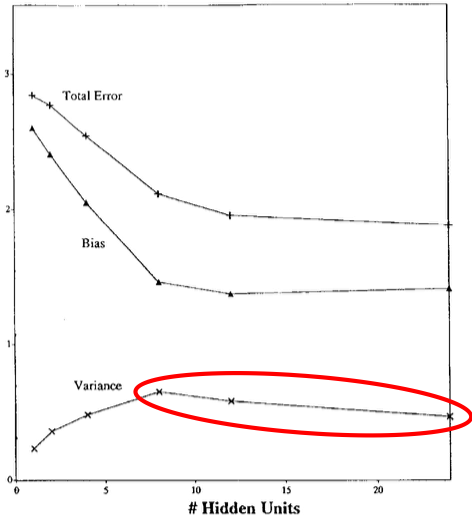Geman et al. (1992) then maintain their claim that there is a bias-variance tradeoff in network width:

The basic trend is what we expect: bias falls and variance increases with the number of hidden units.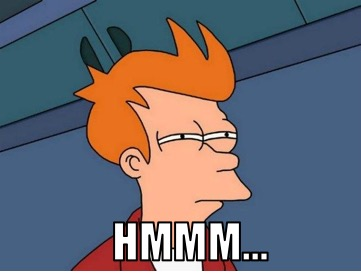They end up noting that the “effects [of the bias-variance tradeoff] are not perfectly demonstrated” and attributing this discrepancy to convergence issues. Geman et al. (1992)’s inclusion of this result where variance decreases for the last 2/3 of the plot, despite it running counter to their claim, is great scholarship. This conflict between their result and their claim does not prevent this paper from being highly influential. This paper has over 3700 citations, over 180 of which were in 2019.

### Test Error Analysis is Not Sufficient

It is time to revisit the bias-variance tradeoff in neural networks. The evidence that Geman et al. (1992) provide for their claims of a bias-variance tradeoff in neural networks is unsatisfying. The recent accumulating evidence that test error does not behave as a U-shaped curve in neural networks seems to suggest that it might not be necessary to trade bias for variance. However, decreasing test error does not imply the lack of a bias-variance tradeoff.

For a clear example where we see both decreasing test error and a tradeoff between bias and variance, consider boosting. In boosting, test error often decreases with the number of rounds (Schapire & Singer, 1999). In spite of this monotonicity in test error in boosting, Bühlmann & Yu (2003, Theorem 1) show that variance does grow, just at an exponentially decaying rate, calling this an “exponential bias-variance tradeoff.” We provide a visualization of this exponential bias-variance tradeoff below: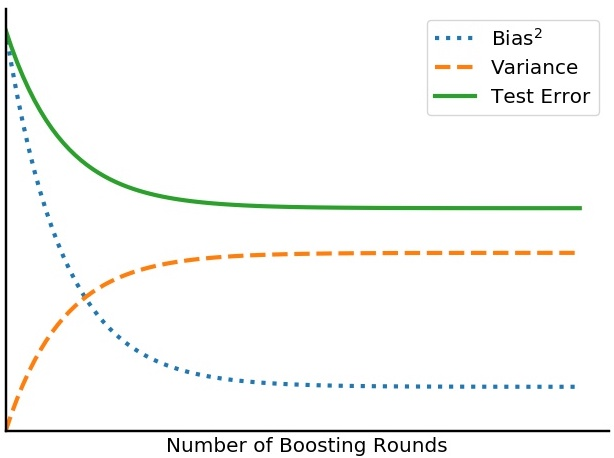This means that a test error analysis is not enough to know if there is a bias-variance tradeoff. In the next section we address this by measuring the specific quantities of interest: bias and variance.

## The Lack of a Tradeoff

### Main Result

So, in 2018 we decided that it is time to revisit the bias-variance tradeoff. In contrast to traditional bias-variance tradeoff wisdom, as exemplified by Geman et al. (1992)’s claims above (1 and 2), we find that both bias and variance decrease with network width, in the over-parameterized regime (Neal et al., 2018):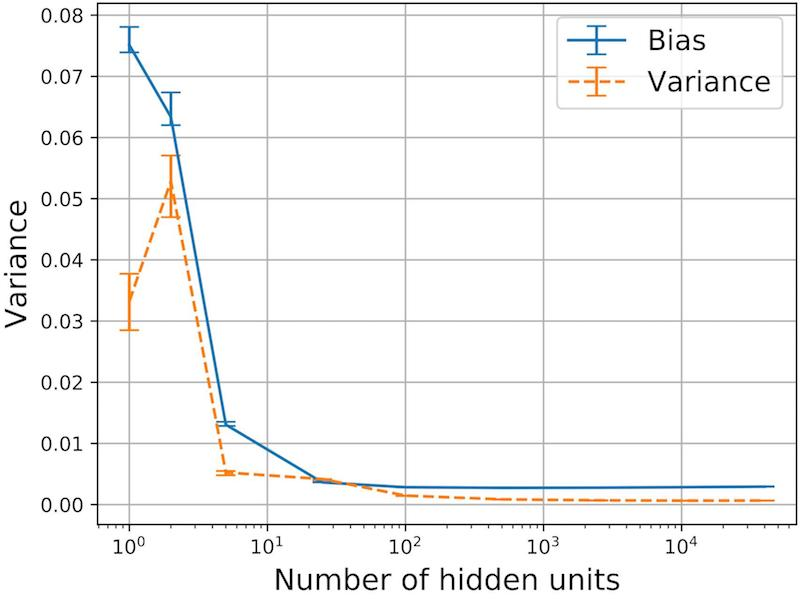We demonstrate these results on CIFAR10, SVHN, MNIST, small MNIST, and a sinusoid regression task. We also find these results are robust to many experimental details such as which gradient-based optimizer is used and which stopping criterion is used.Make the network really wide.

### Why does variance decrease?

This section is an incomplete summary that is meant to give you just enough details to see if you want to read more. Please see A Modern Take on the Bias-Variance Tradeoff in Neural Networks for full details.

In order to better understand why variance decreases with network width in the over-parameterized setting, we introduce a decomposition of the variance, decomposing it into variance due to sampling of the training set (the usual “variance” in the classic bias-variance tradeoff) and variance due to optimization (relevant in non-convex settings with SGD from a random initialization). We also run experiments to estimate these two terms of our variance decomposition.

We provide sufficient conditions that imply decreasing variance with number of parameters (Neal et al., 2018, Section 5). One of the main assumptions is the notion of an “intrinsic dimension” that does not grow with the number of parameters once the network is large enough (Li et al., 2018).

### Double Descent Curve

There is growing evidence that test error acts as the classic U-shaped curve in the under-parameterized regime and monotonically decreases in the over-parameterized regime. Belkin et al. (2019) depict this “double descent” as follows: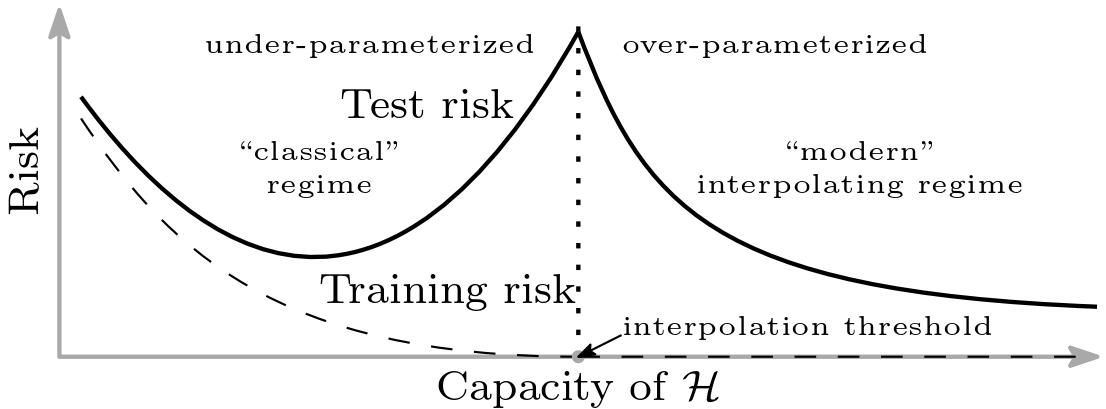Our work, concurrent to the double descent work (Spigler et al., 2018; Geiger et al., 2019; Belkin et al., 2019), focused on the over-parameterized regime. More specifically, rather than searching for a cusp in the test error, we were trying to go far enough out on the x-axis to search for an increase in variance. That said, we did observe humps in the variance, so there is probably a similar phenomenon in variance, which could be an interesting direction for future work. For more discussion on the double descent curve, please see Section 4.4 of my MSc thesis.

## Suggested Updates to Textbooks, Courses, and our Mental Models

Our textbooks, classes, and the majority of the broader machine learning community’s intuition still reflect the classic, rigid tradeoff, missing this newly discovered nuance. Here, we list some proposed updates to the machine learning curriculum. The benefits of these updates range from better informing practitioners on how to think about model selection to helping young machine learning theory researchers have accurate intuitions that will improve their work. These updates are taken from Section 4.5 of my MSc thesis:

1. The bias-variance tradeoff should not be assumed to be universal. For example, we have discussed how there is not a bias-variance tradeoff in the width of neural networks. There might be a similar phenomenon in random forests (Belkin et al., 2019).

2. The bias-variance decomposition does not imply a tradeoff. If we consider a fixed test error, the decomposition does imply a tradeoff between bias and variance. However, in general, test error does not remain fixed as model complexity varies, so this implication does not hold. This lack of implication should be made explicit in textbooks and other learning resources. Currently, the decomposition is often presented in close proximity to the tradeoff as ambiguous evidence for it.

3. Loose bounds should not be interpreted as necessarily predictive of the quantities they bound, unless they have been shown to be. For example, upper bounds on the generalization gap, variance, or estimation error often grow with some measure of model complexity. It is not uncommon to interpret these bounds as confirming our intuition that the bounded quantities (e.g. generalization gap) will grow with model complexity, which supports the bias-variance tradeoff hypothesis. However, many of these bounds are actually negatively correlated with the quantities they are bounding in neural networks (Neyshabur et al., 2017). Of course, if these bounds were tight, these problems would be alleviated (Dziugaite & Roy, 2017).

## Concluding Thoughts

While the concept of the bias-variance tradeoff is useful and accurate sometimes, especially in nonparametric models (see The Bias-Variance Tradeoff), thinking of the tradeoff as universal can be misleading. For example, we show that it is not necessary to trade bias for variance in neural networks (see Main Result). There are several updates that we can make to our textbooks, courses, and mental models that will help better inform practice and help us have accurate intuitions for research.

Although there is a lot of evidence for the double descent curve when increasing neural network width, even in architectures such as ResNets and transformers (Nakkiran et al., 2020), the double descent curve is also not universal. For example, it does not appear to be present in nonparametric models such as k-nearest neighbors and kernel regression. We do not comprehensively know in what models we see the classic U-shaped curve, the double descent curve, or something else. Even within neural networks, there are ways to increase model complexity other than increasing network width; for example, increasing network depth is a natural one (see, e.g., Neal et al. (2018, Appendix C)). The test error curve may very well look different, depending on how model complexity is varied.

Furthermore, measuring the more specific quantities, bias and variance (as opposed to just test error), is important to support claims about the bias-variance tradeoff or lack thereof (see Test Error Analysis is Not Sufficient).

### Acknowledgments

Thanks to Florent Krzakala for pointing out the connection to the historical measurements of the charge of the electron. Thanks to Ioannis Mitliagkas for recommending I put this connection in the preamble, for recommendations on the general ordering of content, and for many edits. Thanks to Xavier Bouthillier and Sherjil Ozair for helpful feedback on the blog post.

1. Belkin, M., Hsu, D., Ma, S., & Mandal, S. (2019). Reconciling modern machine learning practice and the classical bias-variance trade-off. Proceedings of the National Academy of Sciences.
2. Bühlmann, P., & Yu, B. (2003). Boosting with the L2 Loss: Regression and Classification. Journal of the American Statistical Association.
3. Dziugaite, G. K., & Roy, D. M. (2017). Computing Nonvacuous Generalization Bounds for Deep (Stochastic) Neural Networks with Many More Parameters than Training Data. UAI 2017.